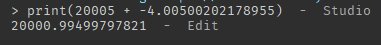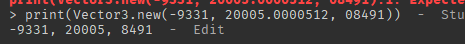# How is this possible? (Vector3 incorrect math operations)

How is this possible?

For context, the Y value in a vector that got printed, should be a decimal. Do Vector3 math operations only work with 3 decimal points max? And is there any way to go around this while having the proper amount of decimals?

It’s called a floating point, basically the computer uses bits and so when doing math, instead of doing it perfectly, when using decimal numbers it uses a floating point to conserve bits

It’s odd that it would treat the floating point as different in the vector. When adding the y values seperately, it works correctly:edit: realised i missed a zero, but the point still stands:So basically, Roblox rounds Vector3 math operations to 3 decimals, and the only way around this is a custom math operation for Vector3.

I don’t think it is exclusive to math operations:No, this is completely wrong. The default tostring() operation for vectors limits the significant digits displayed for clarity. Vectors in roblox are single precision floats, so they always have 23 bits of precision for a given exponent. This is equivalent to about 7 significant decimal digits (~ 23 / log2(10)). You can still run into precision problems if you add small numbers to large ones, starting at about 1 + 0.0000001; or equivalently 10000000 + 1. You can also have “accumulated error” problems if you repeatedly add two numbers, such as angles.

1 Like

This topic was automatically closed 14 days after the last reply. New replies are no longer allowed.